# AVL Tree Insertion | Insertion in AVL Tree

## AVL Tree-

Before you go through this article, make sure that you have gone through the previous article on AVL Trees.

We have discussed-

• AVL trees are self-balancing binary search trees.
• In AVL trees, balancing factor of each node is either 0 or 1 or -1.

In this article, we will discuss insertion in AVL tree.

## Insertion in AVL Tree-

 Insertion Operation is performed to insert an element in the AVL Tree.

To insert an element in the AVL tree, follow the following steps-

• Insert the element in the AVL tree in the same way the insertion is performed in BST.
• After insertion, check the balance factor of each node of the resulting tree.

Read More- Insertion in Binary Search Tree

Now, following two cases are possible-

### Case-01:

• After the insertion, the balance factor of each node is either 0 or 1 or -1.
• In this case, the tree is considered to be balanced.
• Conclude the operation.
• Insert the next element if any.

### Case-02:

• After the insertion, the balance factor of at least one node is not 0 or 1 or -1.
• In this case, the tree is considered to be imbalanced.
• Perform the suitable rotation to balance the tree.
• After the tree is balanced, insert the next element if any.

Also Read- AVL Tree Properties

## Rule-01:

After inserting an element in the existing AVL tree,

• Balance factor of only those nodes will be affected that lies on the path from the newly inserted node to the root node.

## Rule-02:

To check whether the AVL tree is still balanced or not after the insertion,

• There is no need to check the balance factor of every node.
• Check the balance factor of only those nodes that lies on the path from the newly inserted node to the root node.

## Rule-03:

After inserting an element in the AVL tree,

• If tree becomes imbalanced, then there exists one particular node in the tree by balancing which the entire tree becomes balanced automatically.
• To re balance the tree, balance that particular node.

To find that particular node,

• Traverse the path from the newly inserted node to the root node.
• Check the balance factor of each node that is encountered while traversing the path.
• The first encountered imbalanced node will be the node that needs to be balanced.

To balance that node,

• Count three nodes in the direction of leaf node.
• Then, use the concept of AVL tree rotations to re balance the tree.

## Problem-

Construct AVL Tree for the following sequence of numbers-

50 , 20 , 60 , 10 , 8 , 15 , 32 , 46 , 11 , 48

## Solution-

### Step-01: Insert 50### Step-02: Insert 20

• As 20 < 50, so insert 20 in 50’s left sub tree.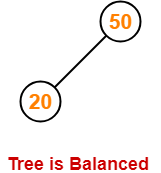### Step-03: Insert 60

• As 60 > 50, so insert 60 in 50’s right sub tree.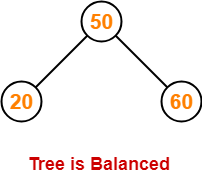### Step-04: Insert 10

• As 10 < 50, so insert 10 in 50’s left sub tree.
• As 10 < 20, so insert 10 in 20’s left sub tree.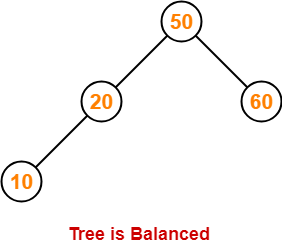### Step-05: Insert 8

• As 8 < 50, so insert 8 in 50’s left sub tree.
• As 8 < 20, so insert 8 in 20’s left sub tree.
• As 8 < 10, so insert 8 in 10’s left sub tree.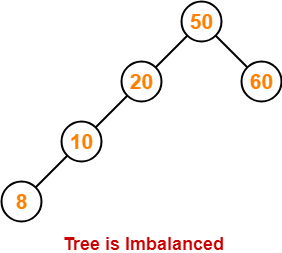To balance the tree,

• Find the first imbalanced node on the path from the newly inserted node (node 8) to the root node.
• The first imbalanced node is node 20.
• Now, count three nodes from node 20 in the direction of leaf node.
• Then, use AVL tree rotation to balance the tree.

Following this, we have-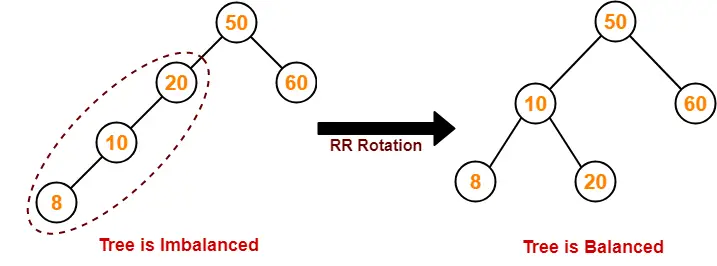### Step-06: Insert 15

• As 15 < 50, so insert 15 in 50’s left sub tree.
• As 15 > 10, so insert 15 in 10’s right sub tree.
• As 15 < 20, so insert 15 in 20’s left sub tree.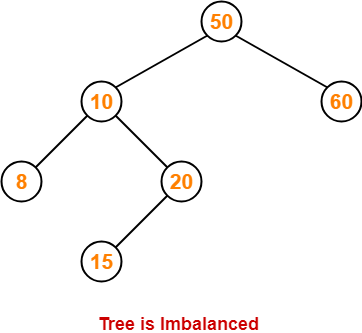To balance the tree,

• Find the first imbalanced node on the path from the newly inserted node (node 15) to the root node.
• The first imbalanced node is node 50.
• Now, count three nodes from node 50 in the direction of leaf node.
• Then, use AVL tree rotation to balance the tree.

Following this, we have-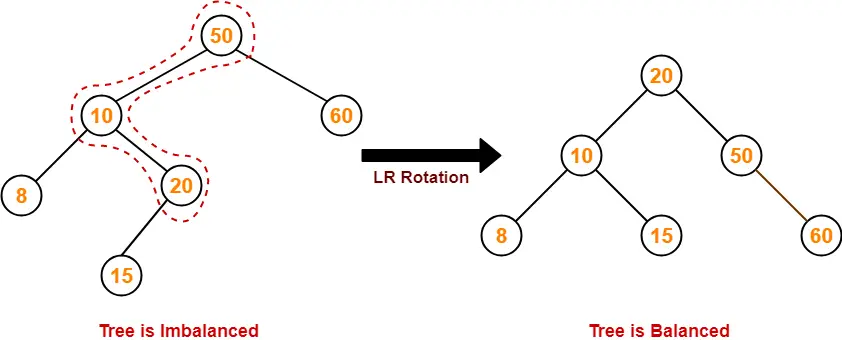### Step-07: Insert 32

• As 32 > 20, so insert 32 in 20’s right sub tree.
• As 32 < 50, so insert 32 in 50’s left sub tree.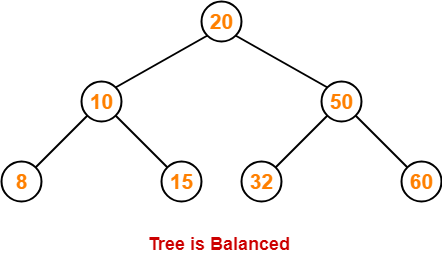### Step-08: Insert 46

• As 46 > 20, so insert 46 in 20’s right sub tree.
• As 46 < 50, so insert 46 in 50’s left sub tree.
• As 46 > 32, so insert 46 in 32’s right sub tree.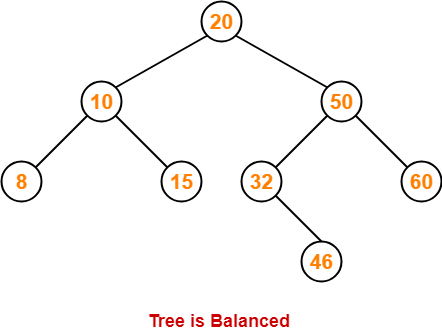### Step-09: Insert 11

• As 11 < 20, so insert 11 in 20’s left sub tree.
• As 11 > 10, so insert 11 in 10’s right sub tree.
• As 11 < 15, so insert 11 in 15’s left sub tree.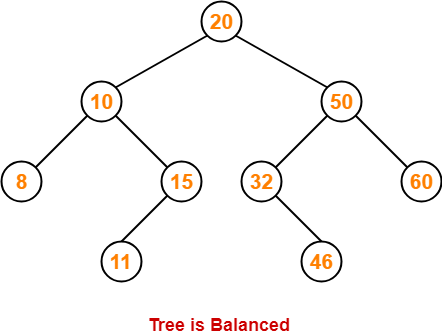### Step-10: Insert 48

• As 48 > 20, so insert 48 in 20’s right sub tree.
• As 48 < 50, so insert 48 in 50’s left sub tree.
• As 48 > 32, so insert 48 in 32’s right sub tree.
• As 48 > 46, so insert 48 in 46’s right sub tree.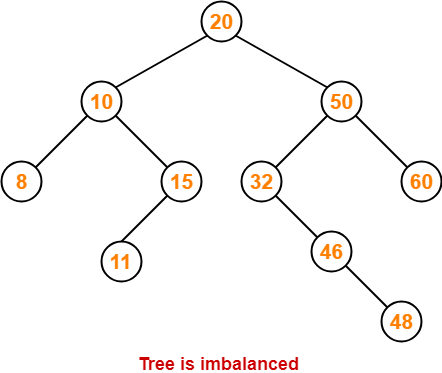To balance the tree,

• Find the first imbalanced node on the path from the newly inserted node (node 48) to the root node.
• The first imbalanced node is node 32.
• Now, count three nodes from node 32 in the direction of leaf node.
• Then, use AVL tree rotation to balance the tree.

Following this, we have-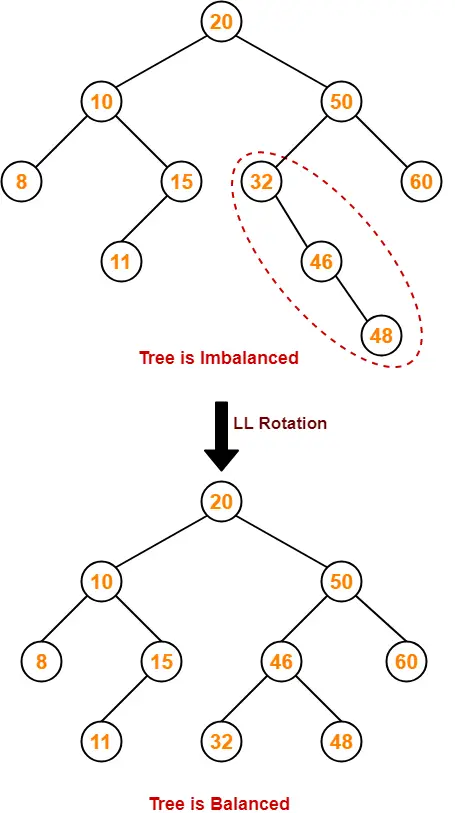This is the final balanced AVL tree after inserting all the given elements.

To gain better understanding of AVL Tree Insertion,

Watch this Video Lecture

Next Article- Heap Data Structure

Get more notes and other study material of Data Structures.

Watch video lectures by visiting our YouTube channel LearnVidFun.

SummaryArticle Name
AVL Tree Insertion | Insertion in AVL Tree
Description
AVL Tree Insertion- Insertion in AVL Tree is performed to insert an element in the AVL tree. Steps to perform insertion in AVL trees. AVL Tree Insertion Example. Insertion in AVL tree is same as insertion in Binary Search Tree with an added step. The tree has to be balanced using AVL tree rotations after performing an insertion operation.
Author
Publisher Name
Gate Vidyalay
Publisher Logo